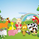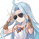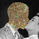# General Filter Estimator-An Experiment on Estimating Everything

‎1046‎ مشاهدة
Introduction

The last indicators i posted where about estimating the least squares moving average , the task of estimating a filter is a funny one because its always a challenge and it require to be really creative. After the last publication of the 1LC-LSMA, who estimate the lsma with 1 line of code and only 3 functions i felt like i could maybe make something more flexible and less complex with the ability to approximate any filter output. Its possible, but the methods to do so are not something that pinescript can do, we have to use another base for our estimation using coefficients, so i inspired myself from the alpha-beta filter and i started writing the code.

Calculation and The Estimation Coefficients

Simplicity is the key word, its also my signature style, if i want something good it should be simple enough, so my code look like that :

• p = length/beta
• a = close - nz (b,close)
• b = nz (b,close) + a/p*gamma

3 line, 2 function, its a good start, we could put everything in one line of code but its easier to see it this way. length control the smoothing amount of the filter, for any filter f(Period) Period should be equal to length and f(Period) = p, it would be inconvenient to have to use a different length period than the one used in the filter we want to estimate (imagine our estimation with length = 50 estimating an ema with period = 100), this is where the first coefficients beta will be useful, it will allow us to leave length as it is. In general beta will be greater than 1, the greater it will be the less lag the filter will have, this coefficient will be useful to estimate low lagging filters, gamma however is the coefficient who will estimate lagging filters, in general it will range around .

We can get loose easily with those coefficients estimation but i will leave a coefficients table in the code for estimating popular filters, and some comparison below.

Estimating a Simple Moving Average

Of course, the boxcar filter, the running mean, the simple moving average , its an easy filter to use and calculate.

For an SMA use the following coefficients :

beta = 2
gamma = 0.5

Our filter is in red and the moving average in white with both length at 50 (This goes for every comparison we will do)

Its a bit imprecise but its a simple moving average , not the most interesting thing to estimate.

Estimating an Exponential Moving Average

The ema is a great filter because its length times more computing efficient than a simple moving average . For the EMA use the following coefficients :

beta = 3
gamma = 0.4

N.B : The EMA is rougher than the SMA , so it filter less, this is why its faster and closer to the price

Estimating The Hull Moving Average

Its a good filter for technical analysis with tons of use, lets try to estimate it ! For the HMA use the following coefficients :

beta = 4
gamma = 0.85

Looks ok, of course if you find better coefficients i will test them and actualize the coefficient table, i will also put a thank message.

Estimating a LSMA

Of course i was gonna estimate it, but this time this estimation does not have anything a lsma have, no moving average, no standard deviation, no correlation coefficient , lets do it.
For the LSMA use the following coefficients :

beta = 3.5
gamma = 0.9

Its far from being the best estimation, but its more efficient than any other i previously made.

Estimating the Quadratic Least Square Moving Average

I doubted about this one but it can be approximated as well. For the QLSMA use the following coefficients :

beta = 5.25
gamma = 1

Another ok estimate, the estimate filter a bit more than needed but its ok.

Jurik Moving Average

Its far from being a filter that i like and its a bit old. For the comparison i will use the JMA provided by @everget described in this article : https://c.mql5.com/forextsd/forum/164/ju...

For the JMA use the following coefficients :

for phase = 0
beta = pow*2 (pow is a parameter in the Jma )
gamma = 0.5

Here length = 50, phase = 0, pow = 5 so beta = 10

Looks pretty good considering the fact that the Jma use an adaptive architecture.

Discussion

I let you the task to judge if the estimation is good or not, my motivation was to estimate such filters using the less amount of calculations as possible, in itself i think that the code is quite elegant like all the codes of IIR filters (IIR Filters = Infinite Impulse Response : Filters using recursion).

It could be possible to have a better estimate of the coefficients using optimization methods like the gradient descent. This is not feasible in pinescript but i could think about it using python or R.

Coefficients should be dependant of length but this would lead to a massive work, the variation of the estimation using fixed coefficients when using different length periods is just ok if we can allow some errors of precision.

I dont think it should be possible to estimate adaptive filter relying a lot on their adaptive parameter/smoothing constant except by making our coefficients adaptive (gamma could be)

So at the end ? What make a filter truly unique ? From my point of sight the architecture of a filter and the problem he is trying to solve is what make him unique rather than its output result. If you become a signal, hide yourself into noise, then look at the filters trying to find you, what a challenging game, this is why we need filters.

Conclusion

I wanted to give a simple filter estimator relying on two coefficients in order to estimate both lagging and low-lagging filters. I will try to give more precise estimate and update the indicator with new coefficients.

ملاحظات الأخبار: Update

New parameter zeta for smoother filters estimation, allow to estimate filters with low passband ripple. The lower zeta is the smoother the resulting output.

Estimating A 3-Poles Butterworth Filter

In blue a 3-Poles Butterworth filter, in red our filter with beta = 5.5, gamma = 0.5 and zeta = 0 with both period 100.
« Je suis las des cruautés de mes semblables, qui ne sont pas mes pareils.

« Je prendrai l’essor et je m’envolerai vers la mer.

« Je connaîtrai le goût des brises du large. J’entendrai les grands cris de la tempête.

## التعليقاتAlthough I am not famous on very short timeframe trading, but I am still appreciated to all your creation Alexgrover, keep it up on all your creations bro =D
ردICEKI
@ICEKI, Thanks a lot for your support, it is really appreciated as always :)
ردalexgrover
@alexgrover, you're very welcome alex, I love all your creation especially your estimation and predicative indicators etc, keep it up, and look forward to seeing your future items =D
ردwroclai
@wroclai, I'm sorry to hear that i made my explanation confusing and hard to understand, i will try to rectify that on future publications, thanks for your feedback.
ردwroclai
@wroclai, Thanks for your kind words, i will still try to work on the mathematical explanations, i dont want to afraid anyone :)
ردWell, well, well... look who it is. Mr. LSMA knocking out of the park as usual.

The only reason I Have a vague interest in signal processing is because of posts like these. I’ll admit, it’s a little over my head, but that’s in no part because of you.

Keep throwing everything you got at us, because we all love your work. You raise the bar for disseminating marble-dense information
ردchrysopoetics
@chrysopoetics, Thanks a lot for your support :) I think i'm done with the lsma for the times to come. It mean a lot to hear that i can bring interest to dsp (digital signal processing) from my work :)
رد